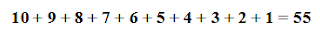### "Sum" Trick

In the book Ten Black Dots book, there are a total of 55 black dots. Normally, to find that answer, you would add the numbers together.But did you know there is an easier way? Take 10 and divide it by 2. That equals 5. Multiply 10 x 5 and you get 50 then add in the 5 which equals 55. Too confusing? Well let's look at it in groups that equal 10.

As illustrated above, 10 is by itself so it is 10. Then if we group the numbers so that each group equals ten, we have four additional sets. All together, we have five groups of ten with five left over which equals 55.   5 x 10 = 50 + 5 = 55

This will work for every sequence of consecutive numbers which begins with one and contains an even set. In other words, sets that contain 2, 4, 6, 8, 10, 12... numbers. Merely divide the largest number by 2; multiply the largest number by the quotient, and then add the quotient.

Example:  14, 13, 12, 11, 10,  9,  8,  7,  6,  5,  4,  3,  2, 1

This will also work for an odd numbered sequence like 11 but the formula or quick trick for finding the sum is a little different. As seen below, we again divide 11 by 2, which 5.5 or rounded up equals 6. Again we group sets of two that equal 11. There are five groups plus 11 by itself so that makes a total of six groups.
Since there are no numbers left by themselves, simply multiply 11 by 6 (the rounded up quotient) to get the sum which is 66.

I love to write a series of consecutive numbers which begin with one on the board, and have the students find the answer using their calculators while I do the math in my head. Of course, they are amazed and swear that I have memorized the answer. I then ask me to give me a series (not off the wall or so large that it would take forever to use the calculator) and again I quickly give them the answer. I then teach them that math trick.

Students love "tricks" like this, but I always burst their bubble by telling them mathematicians are astute people. That's why they are always looking for faster, quicker, and smarter ways to do math!